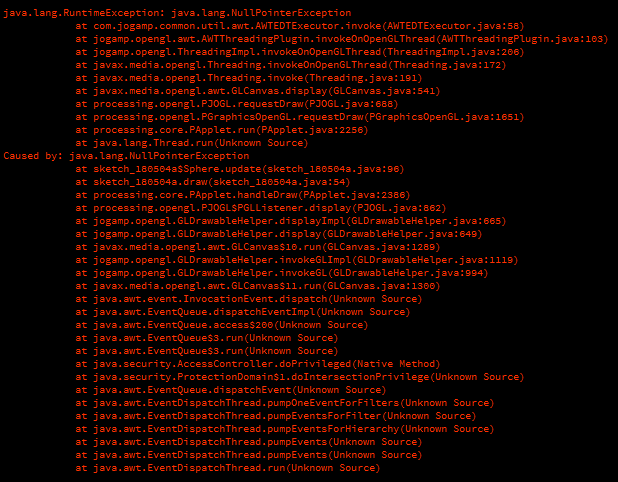#### Howdy, Stranger!

We are about to switch to a new forum software. Until then we have removed the registration on this forum.

# NullPointerException with an unkown source

edited May 2018

Hello !

So today at my school I wanted to play around a bit in Processing while talking to some friends when I stumbled across this issue that I can't seem to make heads or tails of.

Basically, my very simple code is giving out some weird nullpointer that I can't track the source of. Here's the entire batch of code :

Main

``````ArrayList<Sphere> spheres = new ArrayList<Sphere>();

float rot = 0;

void setup()
{
size(500,500,P3D);

lights();

for(int i = 0; i < 10; i++)
{
}
}

void draw()
{
background(50);

if(keyPressed)
{
if(keyCode == LEFT)
{
rot = rot - PI/180;
}
else if(keyCode == RIGHT)
{
rot = rot + PI/180;
}
}

translate(width/2, height/2, 0);
rotateY(rot);

for(int i = 0; i < spheres.size(); i++)
{
spheres.get(i).update();

spheres.get(i).display();
}
}
``````

Sphere

``````class Sphere
{
float x, y, z;

float size;

float xCenter, yCenter, zCenter;

PVector speed;

Sphere()
{
size = 20;

xCenter = 0;
yCenter = 0;
zCenter = 0;

x = random(xCenter - size, xCenter + size);
y = random(yCenter - size, yCenter + size);
z = random(zCenter - size, zCenter + size);
}

void update()
{
if(keyPressed)
{
if(keyCode == UP)
{
speed = new PVector(xCenter - x, yCenter - y, zCenter - z);
}
else if(keyCode == DOWN)
{
speed = new PVector(x - xCenter, y - yCenter, z - zCenter);
}
}

x = x + speed.x;
y = y + speed.y;
z = z + speed.z;
}

void display()
{
translate(x, y, z);

fill(255);
noStroke();

sphere(size);

translate(-x, -y, -z);
}
}
``````

And the error shown in the console is the following (which I can't make sense of because I'm but a highschooler who codes in his free time) :Tagged:

• DEfine PVector speed;

in the class

• edited May 2018 Answer ✓

Until either UP or DOWN is pressed, the Sphere::speed is still `null`! #-o

Initialize the field at the same time you declare it: `final PVector speed = new PVector();`

And rather than keep creating a new PVector object to assign it to Sphere::speed over & over, invoke PVector::set() method. *-:)

That is, replace this: `speed = new PVector(xCenter - x, yCenter - y, zCenter - z);`
With this more efficient code: `speed.set(xCenter - x, yCenter - y, zCenter - z);`